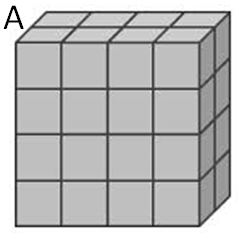Calculating Volume of Rectangular Prisms
starstarstarstarstarstarstarstarstarstar
4.5 (2 ratings)
by Jennifer Miller
| 6 Questions
1
1 pt
How many cubes are in the BASE of the prism?2
1 pt
How many layers are in the prism?3
1 pt
If there are 8 cubes in one layer, and there are four layers, how many cubes in the entire prism?
4
1 pt
Volume is
A the flat side of a rectangular prism
B the amount of cubic units that can fit into a container
C the number of square units that fit on the base of a figure
D how many lengths go into a prism
5
1 pt
To find the volume of a prism, we use the formula V = Bh.
Why is this the same as multiplying L x W x H in a recangular prism?

6
1 pt
BONUS:
Find the total volume of the complex figure. What strategies did you use to help you?